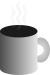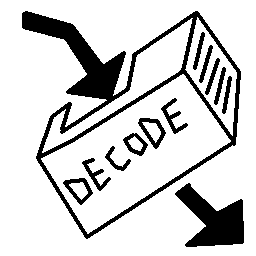#Coffee SpaceListen:

## In-Place DecodePreview Image

When working with memory critical code in C, you may find you don't want to `malloc()` some addition space to perform some simple decoding. In this case, we can choose to do it in-place.

To do this, we need to make some assumptions about the nature of the decoding:

1. Replacing characters does not affect the decode - We assume that once we replace the encoded characters with the decoded characters, that it does not affect the rest of the decoding process.
2. The decoded string is always the same size or smaller than the encoded string - If not, then we will need a larger memory allocation and all performance gains may be lost.
3. The array is not being used by some other process - If something else is assuming it is still encoded after, at the very least we need to take a copy.

I couldn't find anything online, so decided to write my own implementation.

### URL Decoding

The following is a URL decoder (otherwise called percent-encoding) for 7-bit ASCII. This code is used for decoding a HTML form:

```0001 void inplace_decode(char* s){
0002   int z = 0;
0003   int x = -1;
0004   while(s[++x + z] != '\0'){
0005     /* Convert characters in place */
0006     if(s[x + z] == '+'){
0007       s[x] = ' ';```

A `+` character represents a space.

```0008     }else if(s[x + z] == '%'){
0009       /* Check if we meet end of string in next two characters */
0010       if(s[x + z + 1] == '\0' || s[x + z + 2] == '\0'){
0011         break;
0012       }
0013       /* Convert from hex to character */
0014       char h = s[x + z + 1];
0015       h -= h >= '0' && h <= '9' ? '0' : (h >= 'A' && h <= 'F' ? 'A' - 10 : h);
0016       char l = s[x + z + 2];
0017       l -= l >= '0' && l <= '9' ? '0' : (l >= 'A' && l <= 'F' ? 'A' - 10 : l);
0018       s[x] = (h << 4) | l;
0019       /* Setup shift value */
0020       z += 2;```

Decode `%` encoded characters. We don't care exactly what gets decoded for now as we will make sure it looks sane later.

```0021     }else{
0022       s[x] = s[x + z];
0023     }```

Allow un-encoded characters through.

```0024     /* Replace certain characters */
0025     if(s[x] == '<') s[x] = '{';
0026     if(s[x] == '>') s[x] = '}';```

Ensure we don't allow HTML specific characters to get through.

```0027     /* Remove illegal characters */
0028     if((s[x] < ' ' && s[x] != '\n' && s[x] != '\r') || s[x] > '~'){
0029       s[x] = '#';
0030     }
0031   }```

Replace illegal un-printable characters with a `#`.

```0032   s[x] = '\0';
0033 }```

Finally, ensure the data in NULL terminated.

Should you use this in production code? Most definitely not. Could you use this in a spicy homebrew hacked project? Hell yeah!

If anybody plans to use this for some serious projects, most definitely stress-test your code through a fuzzer or something. There are quite a few edge cases to consider!